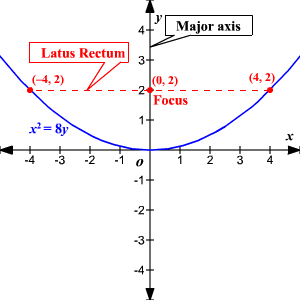# Latus Rectum

The latus rectum of a conic section is the chord (line segment) that passes through the focus, is perpendicular to the major axis and has both endpoints on the curve.The length of the latus rectum is determined differently for each conic.

The length of the parabola ’s latus rectum is equal to four times the focal length.

In an ellipse , it is twice the square of the length of the conjugate (minor) axis divided by the length of the transverse (major) axis.

In a circle , the latus rectum is always the length of the diameter .

In a hyperbola , it is twice the square of the length of the transverse axis divided by the length of the conjugate axis.